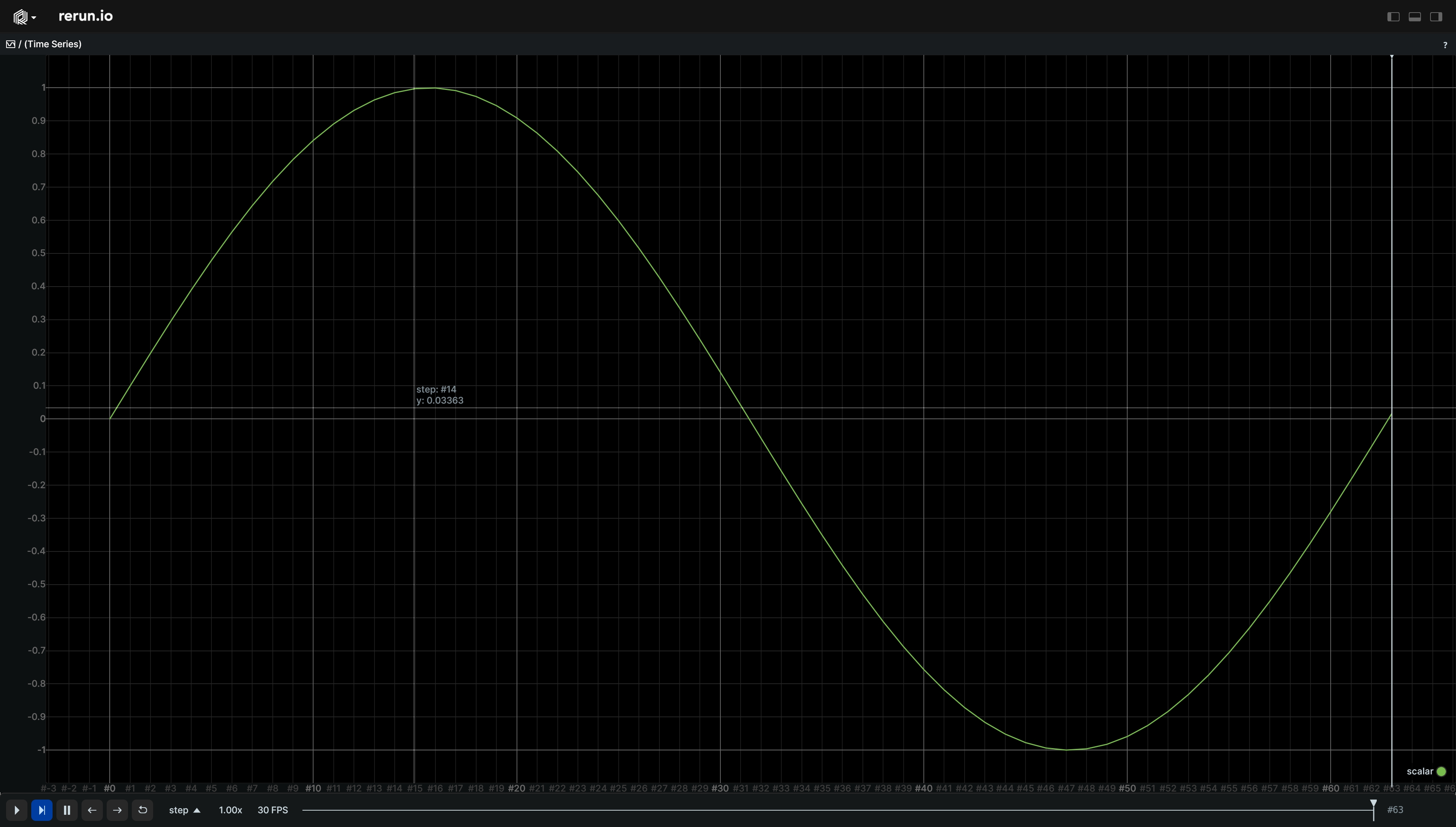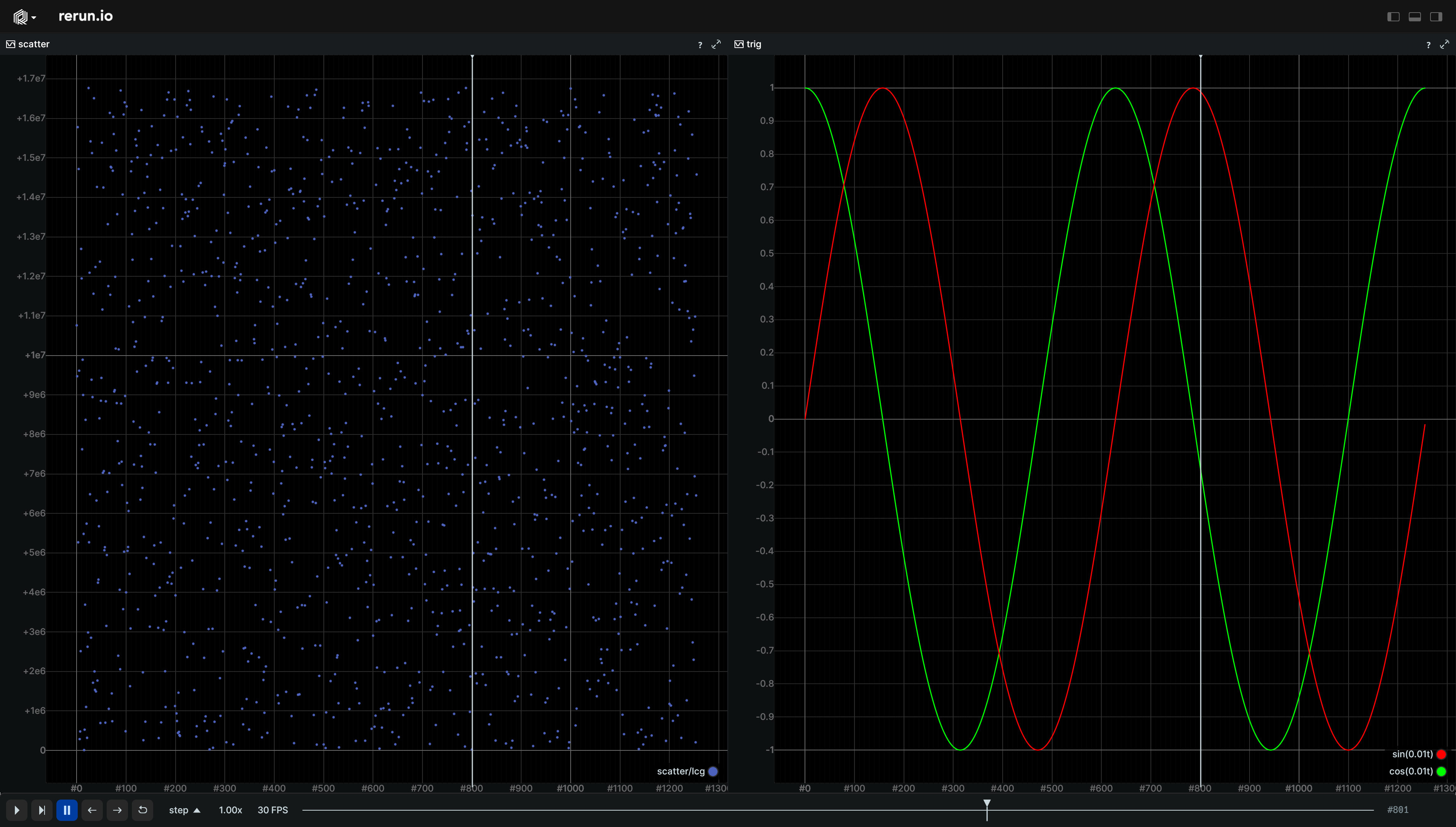# TimeSeriesScalar

Log a double-precision scalar that will be visualized as a time-series plot.

The current simulation time will be used for the time/X-axis, hence scalars cannot be timeless!

## Components

Required: `Scalar`

Recommended: `Radius`, `Color`

Optional: `Text`, `ScalarScattering`

## Examples

### Simple line plot

``````"""Log a scalar over time."""
import math

import rerun as rr

rr.init("rerun_example_scalar", spawn=True)

for step in range(0, 64):
rr.set_time_sequence("step", step)
rr.log("scalar", rr.TimeSeriesScalar(math.sin(step / 10.0)))
``````### Multiple time series plots

``````"""Log a scalar over time."""

from math import cos, sin, tau

import numpy as np
import rerun as rr

rr.init("rerun_example_scalar_multiple_plots", spawn=True)
lcg_state = np.int64(0)

for t in range(0, int(tau * 2 * 100.0)):
rr.set_time_sequence("step", t)

# Log two time series under a shared root so that they show in the same plot by default.
rr.log("trig/sin", rr.TimeSeriesScalar(sin(float(t) / 100.0), label="sin(0.01t)", color=[255, 0, 0]))
rr.log("trig/cos", rr.TimeSeriesScalar(cos(float(t) / 100.0), label="cos(0.01t)", color=[0, 255, 0]))

# Log scattered points under a different root so that they shows in a different plot by default.
lcg_state = (1140671485 * lcg_state + 128201163) % 16777216  # simple linear congruency generator
rr.log("scatter/lcg", rr.TimeSeriesScalar(lcg_state, scattered=True))
``````